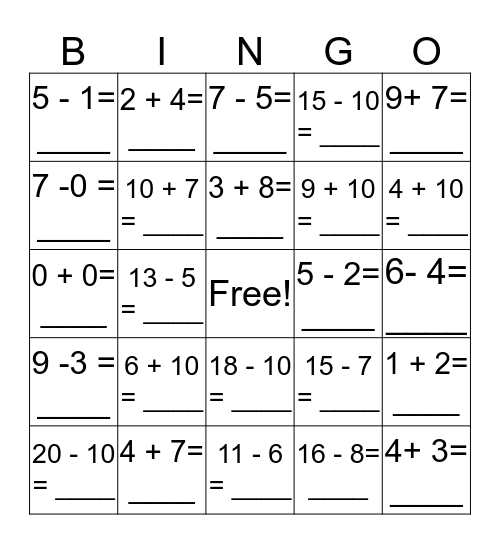This bingo card has a free space and 30 words: 4 + 7= ____, 6 - 6= ____, 13 - 5 = ____, 13 - 7 = ____, 5 - 1= ____, 7 - 5= ____, 10 + 7 = ____, 18 - 10 = ____, 5 - 2= ____, 11 - 6 = ____, 6- 4= ____, 4+ 3= ____, 16 - 8= ____, 5+ 6= ____, 4 + 10 = ____, 0+ 4= ____, 15 - 7 = ____, 9 -3 = ____, 6 + 10 = ____, 9+ 7= ____, 0 + 0= ____, 10 + 8 = ____, 2 + 4= ____, 9 + 10 = ____, 7 -0 = ____, 15 - 10 = ____, 1 + 2= ____, 20 - 10 = ____, 3 + 8= ____ and 7 -1 = ____.

## Play Online